Next: Exercises Up: Incompressible Inviscid Flow Previous: Kelvin Circulation Theorem

# Irrotational Flow

Flow is said to be irrotational when the vorticityhas the magnitude zero everywhere. It immediately follows, from Equation (4.77), that the circulation around any arbitrary loop in an irrotational flow pattern is zero (provided that the loop can be spanned by a surface that lies entirely within the fluid). Hence, from Kelvin's circulation theorem, if an inviscid fluid is initially irrotational then it remains irrotational at all subsequent times. This can be seen more directly from the equation of motion of an inviscid incompressible fluid which, according to Equations (1.39) and (1.79), takes the form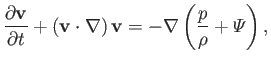(4.82)

because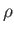is a constant. However, from Equation (A.171),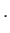(4.83)

Thus, we obtain(4.84)

Taking the curl of this equation, and making use of the vector identities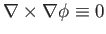[see Equation (A.176)],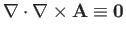[see Equation (A.173)], as well as the identity (A.179), and the fact thatin an incompressible fluid, we obtain the vorticity evolution equation(4.85)

Thus, if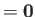, initially, then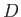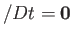, and, consequently,at all subsequent times.

Suppose thatis a fixed point, andan arbitrary movable point, in an irrotational fluid. Letandbe joined by two different paths,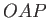and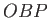(say). It follows that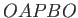is a closed curve. Because the circulation around such a curve in an irrotational fluid is zero, we can write(4.86)

which implies that(4.87)

(say). It is clear that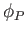is a scalar function whose value depends on the position of(and the fixed point), but not on the path taken betweenand. Thus, ifis the origin of our coordinate system, andan arbitrary point whose position vector is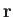, then we have effectively defined a scalar field.

Consider a pointthat is sufficiently close tothat the velocityis constant along. Letbe the position vector ofrelative to. It then follows that (see Section A.18)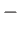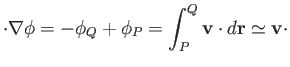(4.88)

The previous equation becomes exact in the limit that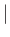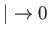. Becauseis arbitrary (provided that it is sufficiently close to), the direction of the vectoris also arbitrary, which implies that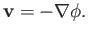(4.89)

We, thus, conclude that if the motion of a fluid is irrotational then the associated velocity field can always be expressed as minus the gradient of a scalar function of position,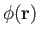. This scalar function is called the velocity potential, and flow which is derived from such a potential is known as potential flow. Note that the velocity potential is undefined to an arbitrary additive constant.

We have demonstrated that a velocity potential necessarily exists in a fluid whose velocity field is irrotational. Conversely, when a velocity potential exists the flow is necessarily irrotational. This follows because [see Equation (A.176)]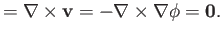(4.90)

Incidentally, the fluid velocity at any given point in an irrotational fluid is normal to the constant-surface that passes through that point.

If a flow pattern is both irrotational and incompressible then we have(4.91)

and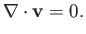(4.92)

These two expressions can be combined to give (see Section A.21)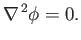(4.93)

In other words, the velocity potential in an incompressible irrotational fluid satisfies Laplace's equation.

According to Equation (4.84), if the flow pattern in an incompressible inviscid fluid is also irrotational, so thatand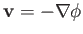, then we can write(4.94)

which implies that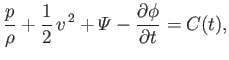(4.95)

where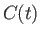is uniform in space, but can vary in time. In fact, the time variation ofcan be eliminated by adding the appropriate function of time (but not of space) to the velocity potential,. Note that such a procedure does not modify the instantaneous velocity fieldderived from. Thus, the previous equation can be rewritten(4.96)

whereis constant in both space and time. Expression (4.96) is a generalization of Bernoulli's theorem (see Section 4.3) that takes non-steady flow into account. However, this generalization is only valid for irrotational flow. For the special case of steady flow, we get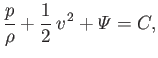(4.97)

which demonstrates that for steady irrotational flow the constant in Bernoulli's theorem is the same on all streamlines. (See Section 4.3.)Next: Exercises Up: Incompressible Inviscid Flow Previous: Kelvin Circulation Theorem
Richard Fitzpatrick 2016-03-31